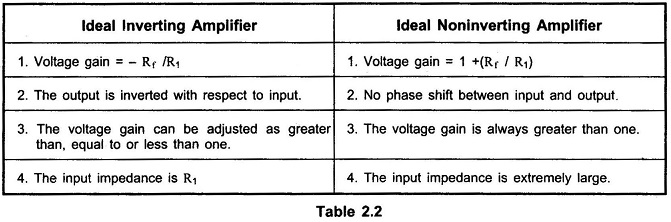## Non Inverting Amplifier using Op Amp:

An amplifier which amplifies the input without producing any phase shift between input and output is called Noninverting Amplifier. The basic circuit diagram of a Non Inverting Amplifier using Op Amp is shown in the Fig. 2.20. The input is applied to the noninverting input terminal of the op-amp.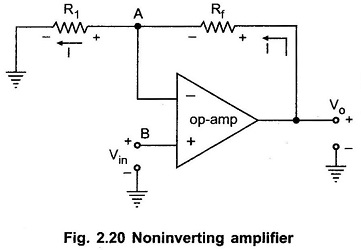Let us derive the expression for its closed loop voltage gain.

The node B is at potential Vin, hence the potential of point A is same as B which is Vin , from the concept of virtual share.From the output side we can write,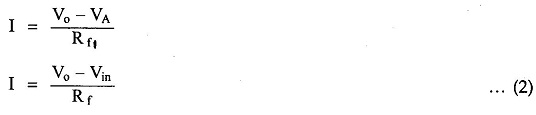At the inverting terminal,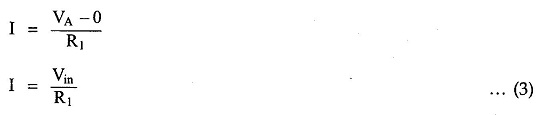Entire current passes through R1 as input current of op-amp is zero.

Equating equations (2) and (3),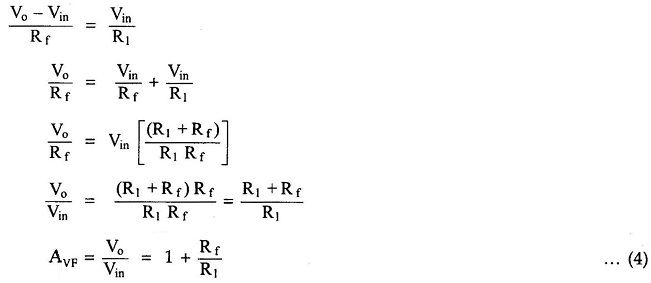The positive sign indicates that there is no phase shift between input and output. The input and output waveforms are shown in the Fig. 2.21.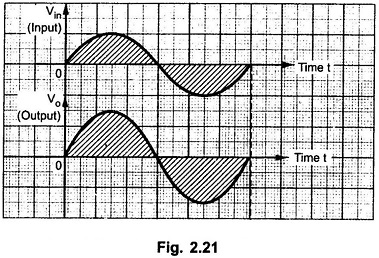#### Difference between inverting and non inverting amplifier:

The Table 2.2 provides the comparison between inverting and noninverting amplifier using op-amp circuits.